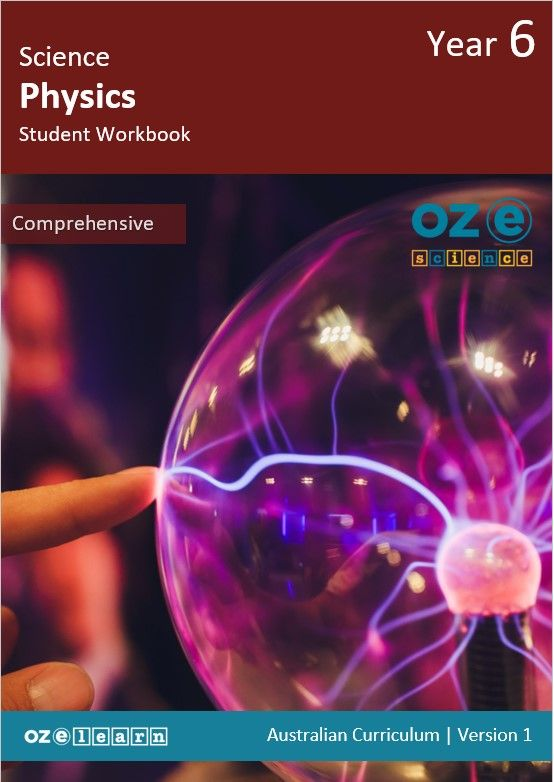## Oz-e-science Years F–6

Physics Comprehensive Year 6### Overview

Physics Comprehensive Year 6 is a Physical Sciences curriculum program combining explicit instruction pedagogy and alignment to the Australian Curriculum Science Understanding:
• Electrical energy can be transferred and transformed in electrical circuits and can be generated from a range of sources. (ACSSU097)

Note:
This curriculum program is currently being updated to align with the Australian Curriculum 9.0, updated versions will be released in early 2024.
Learning Objectives
In Lessons 1 to 15, students learn about:
1. the different types of energy.
2. how energy can be transferred from one place to another.
3. how one kind of energy can be transformed into a different kind of energy.
4. electrical energy and the scientists who added to our knowledge of it.
5. electric currents and static electricity.
6. conductors and insulators.
7. the parts that make up an electrical circuit.
8. electrical diagrams.
9. four common sources of energy that are transformed to generate electrical energy.
10. fossil fuels as sources of energy that are transformed to generate electrical energy.
11. nuclear energy as a source of energy that is transformed to generate electrical energy.
12. renewable energy sources that are transformed to generate electrical energy.
13. investigating which renewable energy source would be best for their town.
14. how to analyse and evaluate their research to find out which renewable energy source would be best for their town.
15. presenting their findings about which renewable energy source would be the best for their town.
Success Criteria
• Explain what energy is.
• Describe six different types of energy.
• Observe energy forms in the school.
• Describe examples of how energy is transferred.
• Represent energy transfer using everyday items.
• Describe examples of how energy is transformed.
• Sort examples of energy transfer and transformation in a card game.
• Explain how electrical energy is generated.
• Describe how four scientists added to our understanding of electrical energy.
• Investigate how to generate electrical energy using a fruit battery.
• Explain how electrical energy moves as static electricity.
• Explain how electrical energy moves as an electric current.
• Investigate the effect of static electricity on different objects.
• Explain what makes a material a conductor or an insulator.
• Identify materials as insulators or conductors using a simple circuit.
• Explain what an electrical circuit is.
• Identify a complete circuit.
• Build a simple circuit.
• Explain what electrical diagrams are used for.
• Describe the different symbols used in electrical diagrams.
• Represent a circuit with a labelled diagram.
• Identify four common sources of energy that are transformed to generate electrical energy.
• Investigate different types of energy used to power a model car.
• Identify three fossil fuels that are used to generate electrical energy.
• Explain how fossil fuels contribute to climate change.
• Investigate which countries use the most energy generated from fossil fuels.
• Identify the sources of nuclear energy that are transformed to generate electrical energy.
• Explain the advantages and disadvantages of using nuclear energy to generate electricity.
• Identify the sources of renewable energy that are transformed to generate electrical energy.
• Explain the advantages and disadvantages of using renewable energy to general electricity.
• Represent the advantages of renewable energy on a poster.
• Conduct research to understand which renewable energy sources might be best for the town.
• Analyse and evaluate the results of the research.
• Form a hypothesis about which renewable energy to use in the town.
• Communicate which renewable energy they would use in their town.
Resources
Progress tests
Progress tests are conducted after every second lesson, allowing teachers to monitor student understanding of the concepts taught over the past two lessons and to identify where reteaching is needed.  The Teaching Guide contains the testing questions, and the Student Workbook has a section where students write their answers and score themselves.

Structured Research Activity
The Structured Research Activity (SRA) for this unit is: Students investigate renewable energy sources, identifying which source would be best for their town and presenting their findings to the class.  The SRA takes place over two lessons so students can apply the Science Understanding and Science Inquiry Skills covered in the unit.  Teachers use the Guide to Making Judgements, which is included in the Teaching Guide, to mark the SRA.

End-of-unit assessment
The last lesson is the end-of-unit assessment, which has a variety of question formats (e.g. label the diagram, circle the correct answer) to assess student mastery of content from the unit.  The end-of-unit assessment is in the Teaching Guide. Teachers copy the assessment and distribute to students at testing time.
Explicit instruction for Science
Oz-e-science uses a teaching approach and curriculum that uses explicit teaching of content knowledge of science and explicit teaching and practical scientific inquiry using the Scientific Method. Read more about pedagogy here.

## Recommended Units

\Learnworlds\Codeneurons\Pages\ZoneRenderers\CourseCards